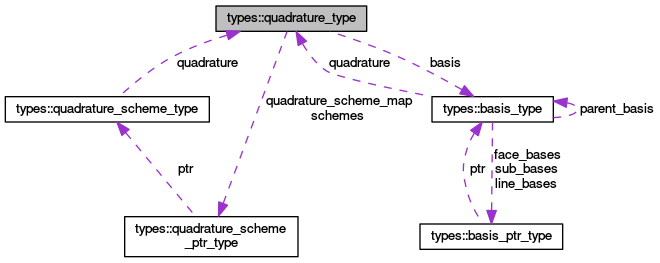OpenCMISS-Iron Internal API Documentation

Contains information on the quadrature to be used for integrating a basis. More...[legend]

## Public Attributes

integer(intg) type
The type of the quadrature. More...

type(basis_type), pointer basis
The pointer back to the basis. More...

integer(intg), dimension(:), allocatable number_of_gauss_xi
NUMBER_OF_GAUSS_XI(ni). For standard Gauss schemes the number of Gauss points to be used in the ni'th xi direction. More...

integer(intg) gauss_order
For simplex Gauss schemes the order of the Quadrature scheme i.e., the order/dimension of the polynomial that can be integrated. More...

QUADRATURE_SCHEME_MAP(scheme_idx). The pointer map to the defined quadrature schemes. The size of array is given by BASIS_ROUTINES::BASIS_NUMBER_OF_QUADRATURE_SCHEME_TYPES. If the quadrature scheme is not defined for the particular type then the array element is NULL. More...

integer(intg) number_of_schemes

SCHEMES(scheme_idx). The array of pointers to the quadrature schemes defined for the basis. scheme_idx must be between 1 and QUADRATURE_TYPE::NUMBER_OF_SCHEMES. More...

logical evaluate_face_gauss =.FALSE.

## Detailed Description

Contains information on the quadrature to be used for integrating a basis.

Definition at line 161 of file types.f90.

## Member Data Documentation

The pointer back to the basis.

Definition at line 163 of file types.f90.

Definition at line 169 of file types.f90.

For simplex Gauss schemes the order of the Quadrature scheme i.e., the order/dimension of the polynomial that can be integrated.

Definition at line 165 of file types.f90.

NUMBER_OF_GAUSS_XI(ni). For standard Gauss schemes the number of Gauss points to be used in the ni'th xi direction.

Definition at line 164 of file types.f90.

Definition at line 167 of file types.f90.

QUADRATURE_SCHEME_MAP(scheme_idx). The pointer map to the defined quadrature schemes. The size of array is given by BASIS_ROUTINES::BASIS_NUMBER_OF_QUADRATURE_SCHEME_TYPES. If the quadrature scheme is not defined for the particular type then the array element is NULL.

Definition at line 166 of file types.f90.